Got a Question? Drop Your Question Right >HERE< - Get an Immediate Response
Or Just Use This Searchbox

# NECO Chemistry Practical 2023 | Questions & Solutions

This post is about NECO Chemistry Practical 2023. We are making the post to help candidates who are finding it difficult to answer NECO Chemistry practical questions. So if you are one of such candidates, ensure that you read through this article. We will show you the best and easy ways to answer NECO Practical Chemistry Alternative A and B questions. You can make a good grade in your Chemistry examination if you pay attention to the formats we will give you here.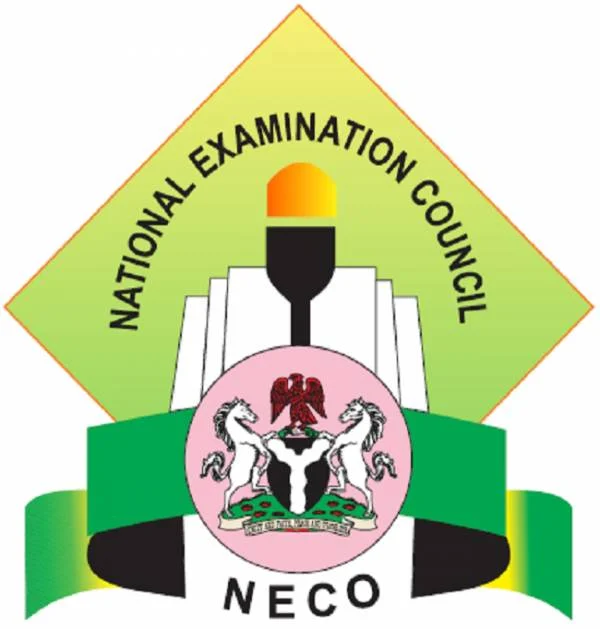Before attempting to answer questions in NECO Chemistry Practicals, it is very important that you understand the  making scheme. The reason is so that you can present your answers in such a way that they meet the requirements. That’s the only guarantee that you will get the full marks allotted to each question you attempt.

Bear in mind that every single procedure is awarded marks. So, ensure you do not skip any step while reporting and presenting your practical results.

The Chemistry Practical question paper will consist of two sections and general Chemistry questions, namely:

1. Quantitative Analysis
2. Qualitative Analysis

These involve titration and test of ions. You will carry out an experiment and report your inference and observation. What you will see will not be far from the NECO Chemistry Specimen given to your school.

Note: Your Chemistry teacher will give you your titration endpoint. It is not the same for every school but within a range.

Do not use the endpoint here. It may vary from your center endpoint but you can follow the calculation procedures.

## NECO Chemistry Practical 2023 Specimen

Below is the 2023 NECO Chemistry Practical specimen…

### Requirements:

(a) One burette (50cm³)

(b) One pippete (20cm³/25cm³). However, all candidates in a center must use pipettes of the same volume.

(c) The usual apparatus and reagents for qualitative work including:
(i) Dilute sodium hydroxide solution
(ii) Dil. ammonia solution
(iii) Dilute hydrochloric acid
(iv) Barium chloride solution
(v) Distilled water
(vi) Red and Blue litmus paper
(vii) Phenolphtalein

(d) Methyl orange

(e) One boiling tube

(f) Five test tubes

(g) Filtration apparatus

(h) Source of heat

### NECO Chemistry Practical 2023 Specimen for Qualitative Analysis

Each candidate should be supplied with the following: with Labels An, Bn, Cn, where ‘n’ is the candidate’s serial number.

(a) 150cm³ of chloride acid solution in a bottle with label “An”. The acid solution which should be the same for all candidates will contain 3.4cm³ of the concentrated hydrochloric acid per dm³ solution.

(b) 150cm³ of sodium hydroxide solution in a bottle with label “Bn”. The solution which should be the same for all candidates will contain 4.0g of sodium hydroxide per dm³ of solution.

(c) One spatulaful of copper (ii) tetraoxosulphate (vi) salt in a specimen bottle labelled “Cn”.

## NECO Chemistry Practical 2023 Practice Questions

The questions below are strictly for practice and not NECO Chemistry Practical 2023 expo.

All your burette readings (initials and final) as well as the size of your pipette must be recorded but no account of experimented procedure is required. All calculations must be done in your booklet.

#### Question 1

A is O.200 moldm3 of HCI. C is a solution containing 14/3g of Na2CO3. x H2O in 500 cm3 of solution.

(a) Put A into the burette and titrate it against 20.0 cm3 or 25.0 cm3 portions of C using methyl orange as indicator. Repeat the titration to obtain Consistent titre values. Tabulate your results and calculate the average volume of A used. The equation for the reaction is: Na2CO3 x H2O + 2HCL(aq) → 2NaCI(aq) + CO2(g) + (x+1) H2O(I).

(b) From your results and the information provided. Calculate the:
(i) concentration of C in moldm-3;
(ii) concentration of C in gdm-3;
(iii) molar mass of Na2CO3, xH2O;
(iv) the value of x in Na2CO3 xH2O. [H = 1.0; C=12.0; O = 16.0; Na =23.0]

Credit will be given for strict adherence to the instruction, for observations precisely recorded and for accurate inferences. All tests, observations and influences must be clearly entered in the booklet in ink at the same time they are made.

#### Question 2

F is a mixture to two inorganic salts. Carry out the following exercise on F. Record your observation and identify any gas(s) evolved. State the conclusions you draw from the result of each test.

(a) Put all of F in a beaker and add about 10cm3 of distilled water. Stir well and filter. Keep the filtrate and the residue.

(b) (i) To about 2cm3 of the filtrate, add NaOH(aq) in drops and then in excess (ii) To another 2cm3 portion of the solution, add a few drops of NHO3(aq) followed by few drops of AgNO3(aq).

(c)(i) Put all the residue into a clean test-tube and add NHO3(aq) followed by few solution from 2(d)(i) add NaOH(aq) in drops and then in excess.

#### Question 3

State what would be observed if the following reactions are carried out in the laboratory:
(i) methyl orange is dropped into a solution of lime juice
(ii) hydrogen sulphide gas is bubbled through iron (III) chloride solution;
(iii) sulphur (IV) oxide gas is bubbled into acidified solution of KMnO4;
(iv) ethanoic acid is added to a solution of K2CO3.

## NECO Chemistry Practical 2023 Answers to Practice Questions

1. Indicator                                 =          Methyl Orange

Volume of the base used        =          25.00cm3

Average Titre              =          1st + 2nd + 3rd/3

=          24.80 + 24.70 + 24.90/3

=          24.80cm3

Alternatively, we can use 2 concordant titres to calculate average titre.

Equation of the reaction: Na2CO3 XH2O + 2HCI(aq) → 2NacI(aq) + CO2(aq) + (x+1)H2O(I)

CAVA/CBVB     =  nA/nB

CA = Molar concentration of HCI(aq) in moldm3

VA = Volume of acid used in cm3 = 24.80cm3

CB = Molar concentration of Na2Cu3 xH2O in moldm3

nA = 2; nB = 1

(b) (i) concentration of C in moldm-3 = ? From the equation of reaction:

CAVA/CBVB     =  nA/nB

CA = 0.200 moldm3     VA = 24.80cm3

CB = ?                          VB = 25.00cm3

Now Substite known values into the equation

0.200 x 24.80/ CB x 25.00 = 2/1

CB = 1×0.200×24.80/2×25.00

C = 0.0992 moldm-3

(ii) Concentration of C in g dm-3 = ?

500cm2 → 14.3 g

1000cm3 →14.3/500 x 10002 g

= 28.6 g dm-3

(iii) Molar mass of Na2CO3 xH2O

Molar conc in moldm-3 = conc in g dm-3 / molar mass

Therefore: Molar mass g mol-1 = conc in g dm-3 / molar conc in moldm-3

= 28.6 g dm-3/0.0992 moldm-3

= 288.3065

Approximately 288 g mol-1

(iv) Value of x in Na2CO3 xH2O?

[H = 1.0, C = 12.0, O = 16.0, Na = 23.0]

Na2CO3 xH2O = 288

2(23) + 12 + 3 (16) + x (2(I) + 16) = 288

46 + 12 + 48 + 18x  = 288

106 + 18x  = 288

18x   = 288 – 106

18x      = 182

x       = 182/18

x       = 10.11

x approximately 10

### Table of Observations, Inferences and Conclusions from Question 2

3 (a)(i) Solution of lime juice turns to pink or red.
(ii) The FeCI3 solution changes colour from brown to green and a yellow deposit.
(iii) The purple colour of the KMnO4 solution turns colorless or decolorised.
(iv) T he solution of K2CO3 reacts with the addition of ethanoic acid to evolve a colorless, odorless gas with bubbling of effervescence.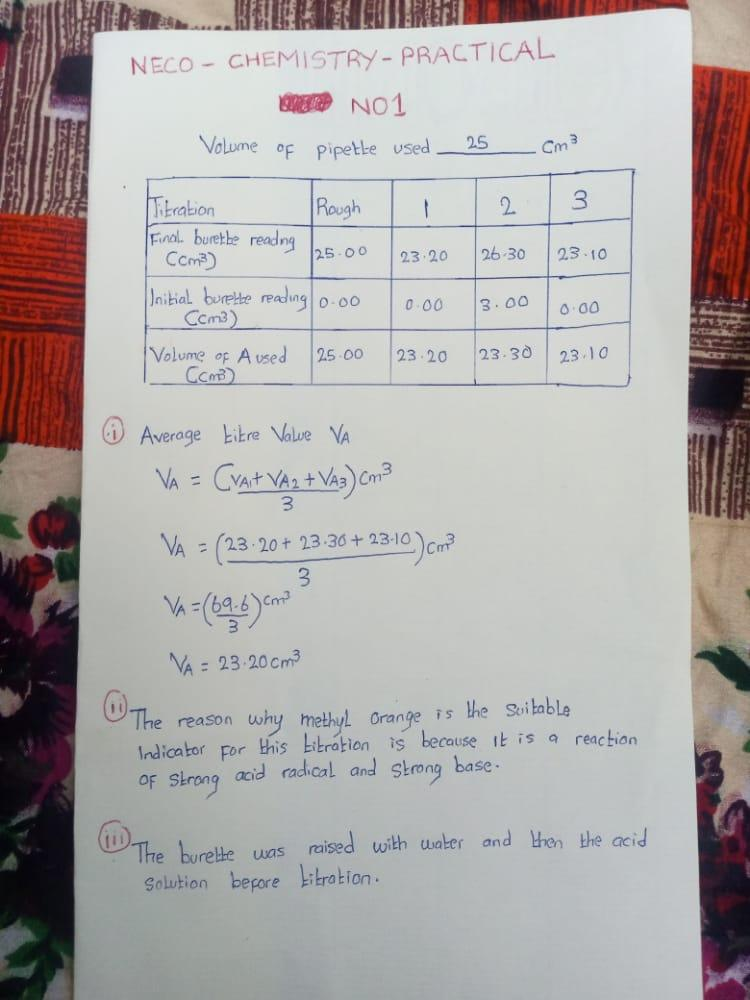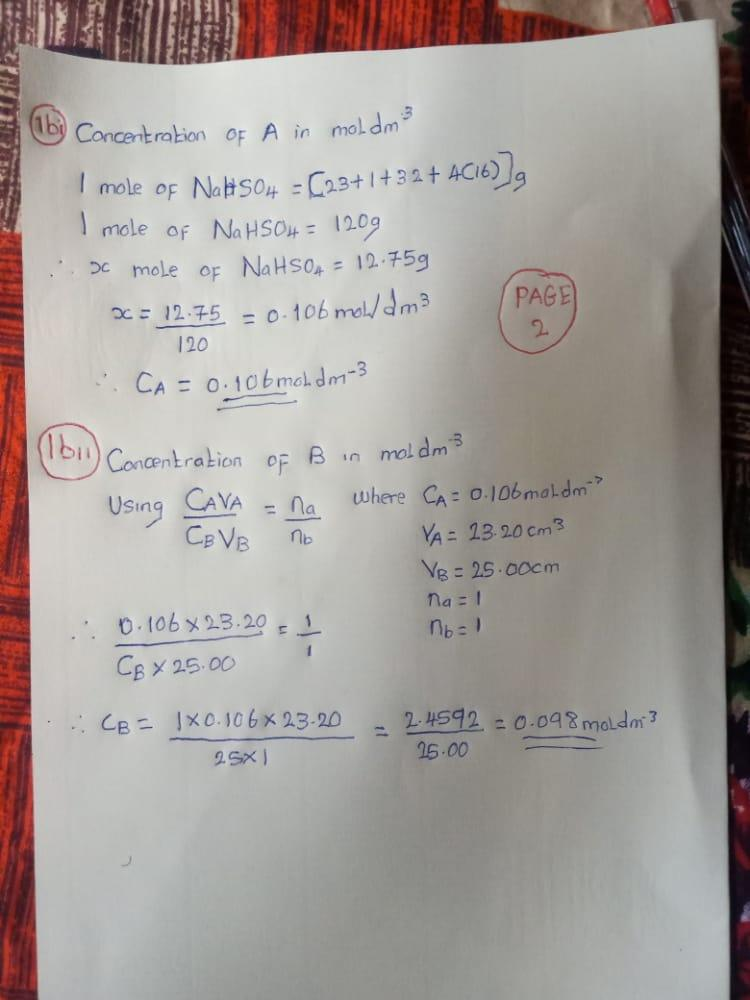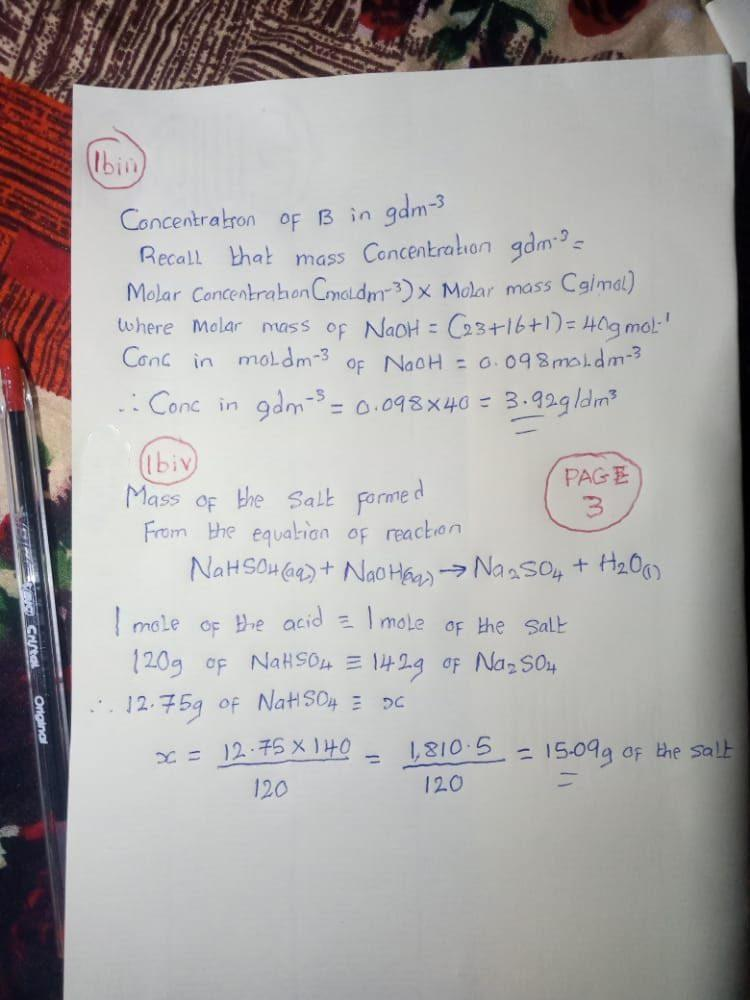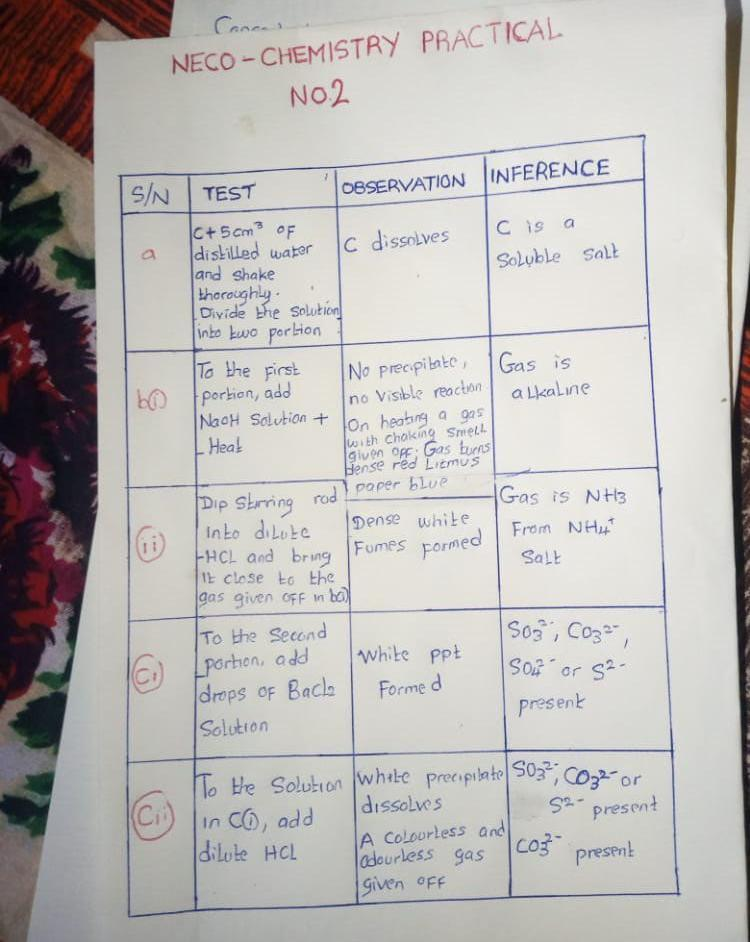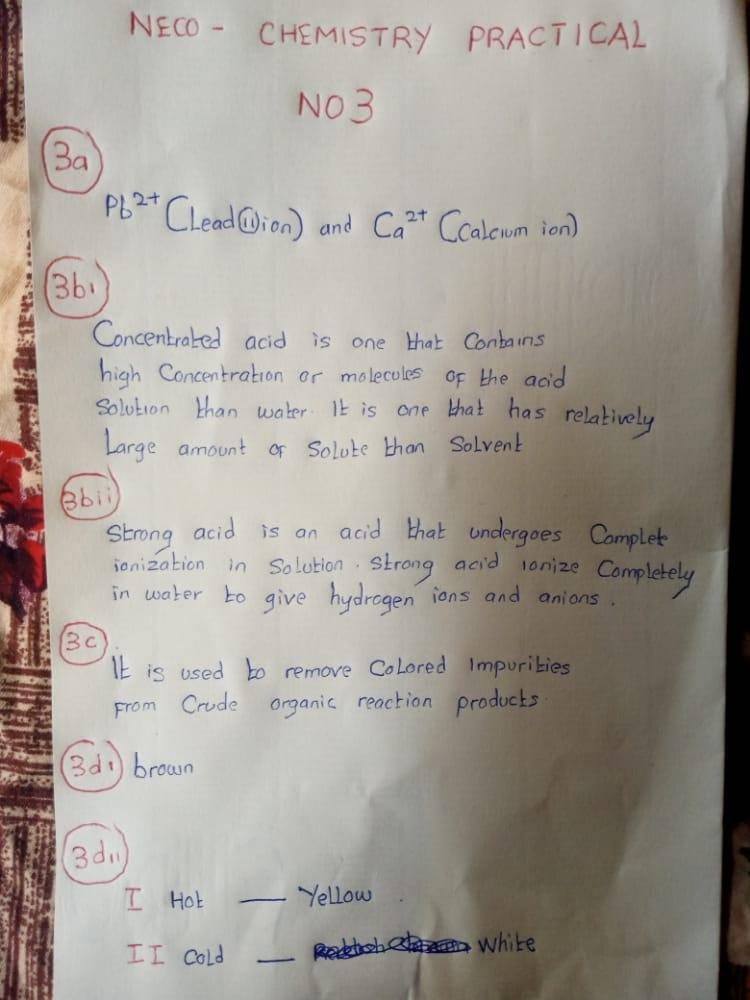If you have any questions about the 2023 NECO Chemistry Practical questions and answers, kindly let us know in the comment box.

Furthermore, kindly help us to reach others with this post. Share with friends on Social Media. Just scroll down to see the Facebook and Twitter and WhatsApp buttons. Thank you so much!

Still Got a Question? Drop Your Question Right HERE 👇👇 and click on Search. Get an Immediate Response...

### Get in touch with us

UNN Aspirants and Students, Join MY UNN DREAMS (MUD)